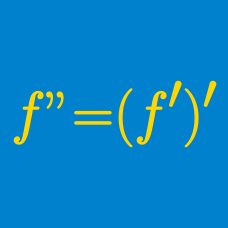Calculus

# Second Order Derivatives

Suppose that $f(x)=xe^{ax+b}, f'(0)=3, f''(0)=6,$ where $a$ and $b$ are constants. What is $a+b?$

What is the second derivative of $f(x)=x^{9}e^x$ with respect to $x$?

If $\displaystyle f(x)=\frac{18}{x},$ what is the value of $\lim_{x \to 1} \frac{f'(x)+18}{x-1}?$

What is the second derivative of $y=(x^2+2x+7)^2?$

$f(x)=xe^{ax+\ln b}$, where $a$ and $b$ are constants. If $f'(0)=7$ and $f''(0)=126$, what is the value of $a+b$?

Details and assumptions

$\ln x$ denotes $\log_e x$.

$f''(x)$ denotes the second derivative of $f(x)$.

×